Do you like the article?
Share it with others -
post a link to it!
Use new possibilities of MetaTrader 5

# Applying The Fisher Transform and Inverse Fisher Transform to Markets Analysis in MetaTrader 5

16 August 2011, 18:32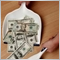5
27 246

### Introduction

The following article presents Fisher Transform and Inverse Fisher Transform applied to financial markets.

The Fisher Transform theory is put into practice by implementing MQL5 version of Smoothed RSI Inverse Fisher Transform indicator presented in October 2010 issue of "Stocks and Commodities" magazine. The indicator profitability is backtested by Expert Advisor that uses signals based on Fisher indicator.

The article is based on J.F.Ehlers books and articles found on the Internet. All references are mentioned at the end of the article.

### 1. Gaussian PDF vs Market Cycles

A common assumption is that prices have normal probability density function.

This means that price deviations from the mean can be described as a well known Gaussian bell: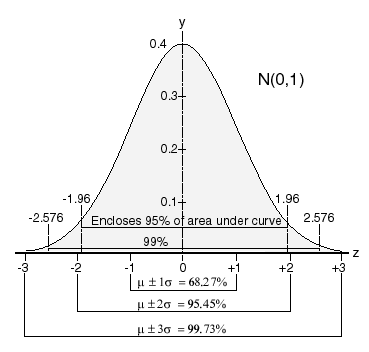Figure 1. Gaussian bell

I mentioned normal probability density function. To fully understand that let's introduce several ideas and math formulas, I hope they will all be understandable for majority of the readers.

Going after Merriam-Webster dictionary probability is defined as

1. The ratio of the number of outcomes in an exhaustive set of equally likely outcomes that produce a given event to the total number of possible outcomes or
2. The chance that a given event will occur.

A random variable is a variable whose value results from a measurement on some type of random process. In our case the random variable is a price of an asset.

Finally, PDF is an acronym for Probability Density Function - a function that describes the probability that a random variable X (again - in our case price) assumes a value in a certain range of possible values. A random variable value that results from a Gaussian distribution or Normal distribution is a probability distribution that is often used to describe real-world random variables that tend to cluster around a single mean value.

Mathematically speaking probability that random variable  X assumes value thal lies in interval [a,b] is defined as integral: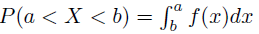This  represents area under the curve f(x) from a to b. Probability is counted from 0 to 100% or from 0 to 1.00, therefore there is a limit that the total area under the f(x) curve must equal 1 (sum of the probabilities):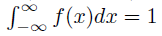Now let's go back to the lower part of Figure 1: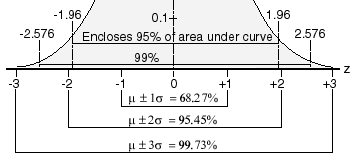Figure 2. Gaussian bell standard deviations

You can see here what percentage of values is under mean +/- 1-3 standard deviations (sigmas). With Gaussian PDF 68.27% of occurences fall within plus/minus one standard deviation from the mean, 95.45% fall within plus/minus two standard deviations and 99.73% fall within plus/minus three standard deviations from the mean.

Do you think that is the case with real market data? Not quite. When we look at market prices we can rather assume that the chart looks like a square wave - after breaching resistance or support levels where large orders are grouped prices tend to rise or fall to the next support/resistance level. That is why market can be modelled with great approximation as a square or sine wave.

Please observe sine plot below:Figure 3. Sine plot

You should notice that in reality most trades are similarily placed near support and resistance levels, which seems quite natural. Now I will plot density plot of a sine wave. You could imagine that we are turning Figure 3 90 degrees to the right and let all circles that make the plot fall to the ground: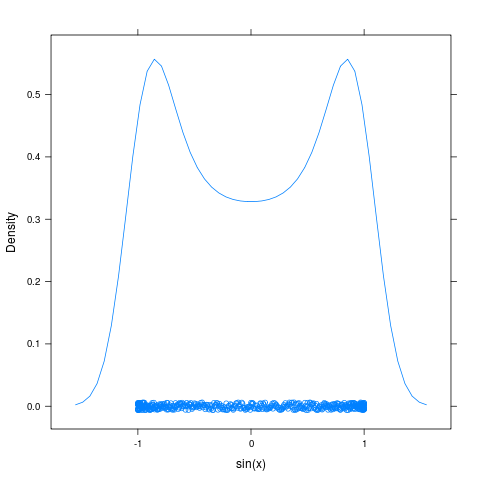Figure 4. Sine curve density plot

You may notice that density is highest on the leftmost and rightmost positions. This seems to be in line with the previous statement that most of the trades are made very close to resistance and support levels. Let's check what percentage of occurences are by drawing a histogram: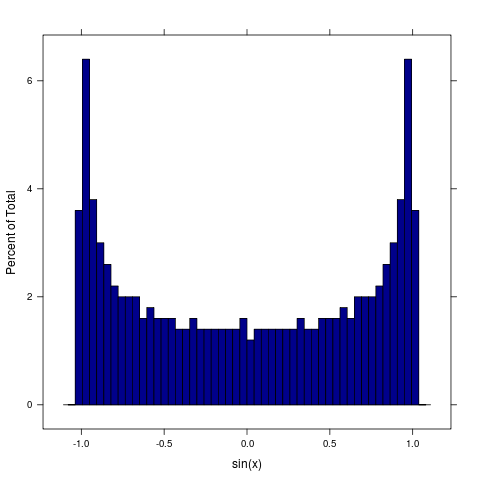Figure 5. Sine curve density histogram

Does it look like a Gaussian bell? Not exactly. First and last three bars appear to have most occurences.

J.F. Ehlers in his book "Сybernetic analysis for stocks and futures" described an experiment where he analysed U.S. T-Bonds over a span of 15 years. He applied a normalized channel 10 bars long and measured the price location within 100 bins and counted the number of times the price was in each bin. The results of this probability distribution closely reminds those of a sine wave.

### 2. Fisher Transform and its application to timeseries

Since we now know that PDF of a market cycle does not remind a Gaussian but rather a PDF of a sine wave and most of the indicators assume that the market cycle PDF is Gaussian we need a way to "correct" that. The solution is to use Fisher Transform. The Fisher transform changes PDF of any waveform to approximately Gaussian.

The equation for Fisher Transform is: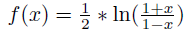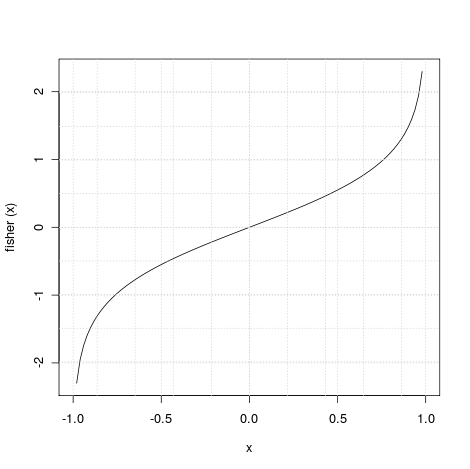Figure 6. Fisher Transform

I mentioned that the output of Fisher transform is aproximately Gaussian PDF. To explain this it is worth to look at the Figure 6.

When the input data is near its mean, the gain is approximately unity (see the chart for |X<0.5|). On the other hand when normalized input approaches either limit the output is greatly amplified (see the chart for 0.5<|x|<1). In practice you might think of growing 'almost Gaussian' tail, when the most deviations occur - this is exactly what happens to the transformed PDF.

How we apply the Fisher Transform to trading? At first, due to |x|<1 constraint, prices must be normalized into this range. When normalized prices are subjected to Fisher Transform the extreme price movements become relatively rare. This means that the Fisher Transform catches those extreme price movements and allows us to trade according to those extremes.

### 3. Fisher Transform in MQL5

Fisher Transform indicator source code is described in Ehlers' book "Cybernetic Analysis for Stocks and Futures".

It has already been implemented in MQL4 and I converted it to MQL5. The indicator uses median prices (H+L)/2, I used iMA() function to extract median prices from history.

At first prices are normalized within 10 bars range and the normalized prices are subjected to the Fisher Transform.

```//+------------------------------------------------------------------+
//|                                              FisherTransform.mq5 |
//|                                      Copyright 2011, Investeo.pl |
//|                                           http://www.investeo.pl |
//+------------------------------------------------------------------+
#property copyright "Copyright 2011, Investeo.pl"
#property link      "http://www.investeo.pl"
#property version   "1.00"
#property indicator_separate_window

#property description "MQL5 version of Fisher Transform indicator"

#property indicator_buffers 4
#property indicator_level1 0
#property indicator_levelcolor Silver
#property indicator_plots 2
#property indicator_type1         DRAW_LINE
#property indicator_color1        Red
#property indicator_width1 1
#property indicator_type2         DRAW_LINE
#property indicator_color2        Blue
#property indicator_width2 1

double Value1[];
double Fisher[];
double Trigger[];

input int Len=10;

double medianbuff[];
int hMedian;

//+------------------------------------------------------------------+
//| Custom indicator initialization function                         |
//+------------------------------------------------------------------+
int OnInit()
{
//--- indicator buffers mapping
SetIndexBuffer(0,Fisher,INDICATOR_DATA);
SetIndexBuffer(1,Trigger,INDICATOR_DATA);
SetIndexBuffer(2,Value1,INDICATOR_CALCULATIONS);
SetIndexBuffer(3,medianbuff,INDICATOR_CALCULATIONS);
ArraySetAsSeries(Fisher,true);
ArraySetAsSeries(Trigger,true);
ArraySetAsSeries(Value1,true);
ArraySetAsSeries(medianbuff,true);

hMedian = iMA(_Symbol,PERIOD_CURRENT,1,0,MODE_SMA,PRICE_MEDIAN);
if(hMedian==INVALID_HANDLE)
{
//--- tell about the failure and output the error code
PrintFormat("Failed to create handle of the iMA indicator for the symbol %s/%s, error code %d",
_Symbol,
EnumToString(PERIOD_CURRENT),
GetLastError());
//--- the indicator is stopped early, if the returned value is negative
return(-1);
}
//---
return(0);
}

//+------------------------------------------------------------------+
//| Custom indicator iteration function                              |
//+------------------------------------------------------------------+
int OnCalculate(const int rates_total,
const int prev_calculated,
const datetime &time[],
const double &open[],
const double &high[],
const double &low[],
const double &close[],
const long &tick_volume[],
const long &volume[],
const int &spread[])
{
//---
int  nLimit=MathMin(rates_total-Len-1,rates_total-prev_calculated);
int copied = CopyBuffer(hMedian,0,0,nLimit,medianbuff);
if (copied!=nLimit) return (-1);
nLimit--;
for(int i=nLimit; i>=0; i--)
{
double price=medianbuff[i];
double MaxH = price;
double MinL = price;
for(int j=0; j<Len; j++)
{
double nprice=medianbuff[i+j];
if (nprice > MaxH) MaxH = nprice;
if (nprice < MinL) MinL = nprice;
}
Value1[i]=0.5*2.0 *((price-MinL)/(MaxH-MinL)-0.5)+0.5*Value1[i+1];
if(Value1[i]>0.9999) Value1[i]=0.9999;
if(Value1[i]<-0.9999) Value1[i]=-0.9999;
Fisher[i]=0.25*MathLog((1+Value1[i])/(1-Value1[i]))+0.5*Fisher[i+1];
Trigger[i]=Fisher[i+1];
}
//--- return value of prev_calculated for next call
return(rates_total);
}
//+------------------------------------------------------------------+
```

Please note that sharp signals are generated.

Signal line is simply Fisher transformed price delayed by one bar: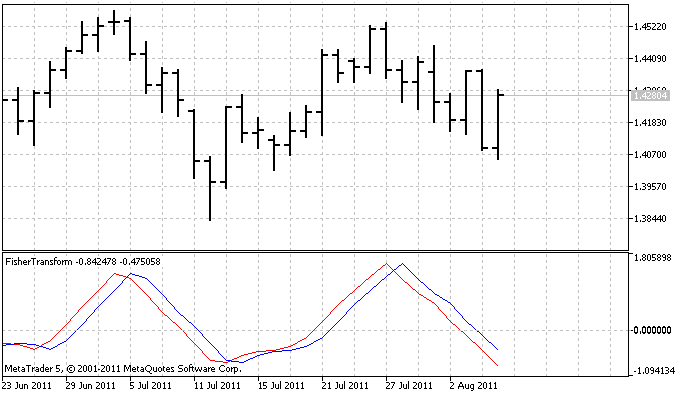Figure 7. Fisher Transform indicator

### 4. Inverse Fisher Transform and its application to cycle indicators

Inverse Fisher Transform equation is obtained by solving Fisher Transform equation for x in terms of y: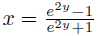,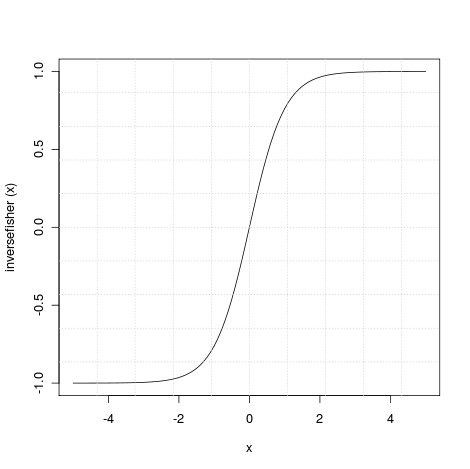Figure 8. Inverse Fisher Transform

The transfer response of this function is inverse of that of the Fisher Transform.

For |x|>2 the input is compressed to not exceeding unity (for negative numbers -1 and for positive +1) and for |x|<1 it is an almost linear relationship which means that ouput has more less the same characteristics as input.

The results is that when Inverse Fisher Transform is applied to properly prepared input data, the output has a big chance to be -1 or +1. This makes the Inverse Fisher Transform perfect to apply it to oscillator indicators. The Inverse Fisher Transform can improve them by giving sharp buy or sell signals.

### 5. Example of Inverse Fisher Transform in MQL5

In order to verify the Inverse Fisher Transform I implemented MQL5 version of Sylvain's Vervoort Smoothed RSI Inverse Fisher Transform indicator presented in October 2010 issue of "Stocks and Commodities" magazine and build a trading signal module and Expert Advisor based on that indicator.

Inverse Fisher Transform indicator has already been implemented for many trading platforms, the source codes are available at traders.com website and MQL5.com Code Base.

Since there was no iRSIOnArray function in MQL5 I added it to the indicator code. The only difference with the original indicator is default RSIPeriod set to 21 and EMAPeriod set to 34 since it behaved better for my settings (EURUSD 1H). You may want to change it to default RSIPeriod 4 and EMAPeriod 4.

```//+------------------------------------------------------------------+
//|                            SmoothedRSIInverseFisherTransform.mq5 |
//|                                      Copyright 2011, Investeo.pl |
//|                                           http://www.investeo.pl |
//+------------------------------------------------------------------+
#property copyright "Copyright 2011, Investeo.pl"
#property link      "http://www.investeo.pl"
#property version   "1.00"
#property indicator_separate_window
#include <MovingAverages.mqh>
#property description "MQL5 version of Silvain Vervoort's Inverse RSI"
#property indicator_minimum -10
#property indicator_maximum 110
#property indicator_buffers 16
#property indicator_level1 12
#property indicator_level2 88
#property indicator_levelcolor Silver
#property indicator_plots 1
#property indicator_type1         DRAW_LINE
#property indicator_color1        LightSeaGreen
#property indicator_width1 2

int                  ma_period=10;             // period of ma
int                  ma_shift=0;               // shift
ENUM_MA_METHOD       ma_method=MODE_LWMA;        // type of smoothing
ENUM_APPLIED_PRICE   applied_price=PRICE_CLOSE;   // type of price

double wma0[];
double wma1[];
double wma2[];
double wma3[];
double wma4[];
double wma5[];
double wma6[];
double wma7[];
double wma8[];
double wma9[];
double ema0[];
double ema1[];
double rainbow[];
double rsi[];
double bufneg[];
double bufpos[];
double srsi[];
double fish[];

int hwma0;

int wma1weightsum;
int wma2weightsum;
int wma3weightsum;
int wma4weightsum;
int wma5weightsum;
int wma6weightsum;
int wma7weightsum;
int wma8weightsum;
int wma9weightsum;

extern int     RSIPeriod=21;
extern int     EMAPeriod=34;

//+------------------------------------------------------------------+
//| Custom indicator initialization function                         |
//+------------------------------------------------------------------+
int OnInit()
{
SetIndexBuffer(0,fish,INDICATOR_DATA);
SetIndexBuffer(1,wma0,INDICATOR_CALCULATIONS);
SetIndexBuffer(2,wma1,INDICATOR_CALCULATIONS);
SetIndexBuffer(3,wma2,INDICATOR_CALCULATIONS);
SetIndexBuffer(4,wma3,INDICATOR_CALCULATIONS);
SetIndexBuffer(5,wma4,INDICATOR_CALCULATIONS);
SetIndexBuffer(6,wma5,INDICATOR_CALCULATIONS);
SetIndexBuffer(7,wma6,INDICATOR_CALCULATIONS);
SetIndexBuffer(8,wma7,INDICATOR_CALCULATIONS);
SetIndexBuffer(9,wma8,INDICATOR_CALCULATIONS);
SetIndexBuffer(10,wma9,INDICATOR_CALCULATIONS);
SetIndexBuffer(11,rsi,INDICATOR_CALCULATIONS);
SetIndexBuffer(12,ema0,INDICATOR_CALCULATIONS);
SetIndexBuffer(13,srsi,INDICATOR_CALCULATIONS);
SetIndexBuffer(14,ema1,INDICATOR_CALCULATIONS);
SetIndexBuffer(15,rainbow,INDICATOR_CALCULATIONS);

ArraySetAsSeries(fish,true);
ArraySetAsSeries(wma0,true);
ArraySetAsSeries(wma1,true);
ArraySetAsSeries(wma2,true);
ArraySetAsSeries(wma3,true);
ArraySetAsSeries(wma4,true);
ArraySetAsSeries(wma5,true);
ArraySetAsSeries(wma6,true);
ArraySetAsSeries(wma7,true);
ArraySetAsSeries(wma8,true);
ArraySetAsSeries(wma9,true);
ArraySetAsSeries(ema0,true);
ArraySetAsSeries(ema1,true);
ArraySetAsSeries(rsi,true);
ArraySetAsSeries(srsi,true);
ArraySetAsSeries(rainbow,true);

PlotIndexSetDouble(0,PLOT_EMPTY_VALUE,0.0);
PlotIndexSetInteger(0,PLOT_DRAW_BEGIN,0);
//--- sets drawing line empty value
PlotIndexSetDouble(0,PLOT_EMPTY_VALUE,0.0);
//--- digits
IndicatorSetInteger(INDICATOR_DIGITS,2);

hwma0=iMA(_Symbol,PERIOD_CURRENT,2,ma_shift,ma_method,applied_price);
if(hwma0==INVALID_HANDLE)
{
//--- tell about the failure and output the error code
PrintFormat("Failed to create handle of the iMA indicator for the symbol %s/%s, error code %d",
_Symbol,
EnumToString(PERIOD_CURRENT),
GetLastError());
//--- the indicator is stopped early, if the returned value is negative
return(-1);
}

return(0);
}
//+------------------------------------------------------------------+
//| Custom indicator iteration function                              |
//+------------------------------------------------------------------+
int OnCalculate(const int rates_total,
const int prev_calculated,
const datetime &time[],
const double &open[],
const double &high[],
const double &low[],
const double &close[],
const long &tick_volume[],
const long &volume[],
const int &spread[])
{
//---
int nLimit;

if(rates_total!=prev_calculated)
{
CopyBuffer(hwma0,0,0,rates_total-prev_calculated+1,wma0);
LinearWeightedMAOnBuffer(rates_total,prev_calculated,0,2,wma0,wma1,wma1weightsum);
LinearWeightedMAOnBuffer(rates_total,prev_calculated,0,2,wma1,wma2,wma2weightsum);
LinearWeightedMAOnBuffer(rates_total,prev_calculated,0,2,wma2,wma3,wma3weightsum);
LinearWeightedMAOnBuffer(rates_total,prev_calculated,0,2,wma3,wma4,wma4weightsum);
LinearWeightedMAOnBuffer(rates_total,prev_calculated,0,2,wma4,wma5,wma5weightsum);
LinearWeightedMAOnBuffer(rates_total,prev_calculated,0,2,wma5,wma6,wma6weightsum);
LinearWeightedMAOnBuffer(rates_total,prev_calculated,0,2,wma6,wma7,wma7weightsum);
LinearWeightedMAOnBuffer(rates_total,prev_calculated,0,2,wma7,wma8,wma8weightsum);
LinearWeightedMAOnBuffer(rates_total,prev_calculated,0,2,wma8,wma9,wma9weightsum);

if(prev_calculated==0) nLimit=rates_total-1;
else nLimit=rates_total-prev_calculated+1;

for(int i=nLimit; i>=0; i--)
rainbow[i]=(5*wma0[i]+4*wma1[i]+3*wma2[i]+2*wma3[i]+wma4[i]+wma5[i]+wma6[i]+wma7[i]+wma8[i]+wma9[i])/20.0;

iRSIOnArray(rates_total,prev_calculated,11,RSIPeriod,rainbow,rsi,bufpos,bufneg);

ExponentialMAOnBuffer(rates_total,prev_calculated,12,EMAPeriod,rsi,ema0);
ExponentialMAOnBuffer(rates_total,prev_calculated,13,EMAPeriod,ema0,ema1);

for(int i=nLimit; i>=0; i--)
srsi[i]=ema0[i]+(ema0[i]-ema1[i]);

for(int i=nLimit; i>=0; i--)
fish[i]=((MathExp(2*srsi[i])-1)/(MathExp(2*srsi[i])+1)+1)*50;
}
//--- return value of prev_calculated for next call
return(rates_total);
}
//+------------------------------------------------------------------+

//+------------------------------------------------------------------+
///                        Calculating RSI
//+------------------------------------------------------------------+
int iRSIOnArray(const int rates_total,const int prev_calculated,const int begin,
const int period,const double &price[],double &buffer[],double &bpos[],double &bneg[])
{
int        i;
//--- check for data
ArrayResize(bneg,rates_total);
ArrayResize(bpos,rates_total);

if(period<=1 || rates_total-begin<period) return(0);
//--- save as_series flags
bool as_series_price=ArrayGetAsSeries(price);
bool as_series_buffer=ArrayGetAsSeries(buffer);
if(as_series_price) ArraySetAsSeries(price,false);
if(as_series_buffer) ArraySetAsSeries(buffer,false);

double diff=0.0;
//--- check for rates count
if(rates_total<=period)
return(0);
//--- preliminary calculations
int ppos=prev_calculated-1;
if(ppos<=begin+period)
{
//--- first RSIPeriod values of the indicator are not calculated
for (i=0; i<begin; i++)
{
buffer[i]=0.0;
bpos[i]=0.0;
bneg[i]=0.0;
}
double SumP=0.0;
double SumN=0.0;
for(i=begin;i<=begin+period;i++)
{
buffer[i]=0.0;
bpos[i]=0.0;
bneg[i]=0.0;
//PrintFormat("%f %f\n", price[i], price[i-1]);
diff=price[i]-price[i-1];
SumP+=(diff>0?diff:0);
SumN+=(diff<0?-diff:0);
}
//--- calculate first visible value
bpos[begin+period]=SumP/period;
bneg[begin+period]=SumN/period;
if (bneg[begin+period]>0.0000001)
buffer[begin+period]=0.1*((100.0-100.0/(1+bpos[begin+period]/bneg[begin+period]))-50);
//--- prepare the position value for main calculation
ppos=begin+period+1;
}
//--- the main loop of calculations

for(i=ppos;i<rates_total && !IsStopped();i++)
{
diff=price[i]-price[i-1];
bpos[i]=(bpos[i-1]*(period-1)+((diff>0.0)?(diff):0.0))/period;
bneg[i]=(bneg[i-1]*(period-1)+((diff<0.0)?(-diff):0.0))/period;
if (bneg[i]>0.0000001)
buffer[i]=0.1*((100.0-100.0/(1+bpos[i]/bneg[i]))-50);
//Print(buffer[i]);
}
//--- restore as_series flags
if(as_series_price) ArraySetAsSeries(price,true);
if(as_series_buffer) ArraySetAsSeries(buffer,true);

return(rates_total);
}
//+------------------------------------------------------------------+
```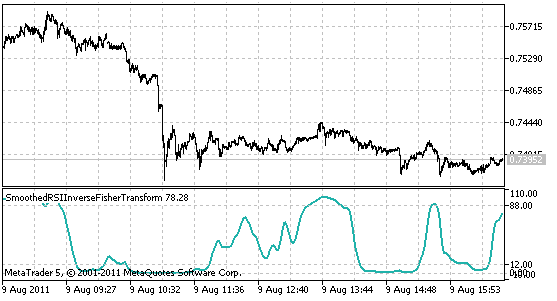Figure 9. Inverse Fisher Transform indicator

Since I only presented transforms equations you might be puzzled on Fisher Transform and Inverse Fisher Transform origins.

When I was gathering materials for writing the article I got interested in how Fisher obtained both transforms but I did not found anything on the Internet.

But I looked at both Fisher Transform and Inverse Fisher Transform and both plots reminded me of a some kind of trigonometric or hyperbolic functions (can you see any similarities?). Since those functions can be derived from Euler's formula and expressed in terms of Euler's number 'e' I went back to calculus books and double checked that: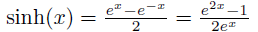,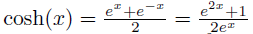,

and since we now that tanh(x) can be obtained by: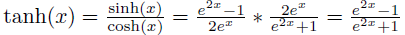,

and...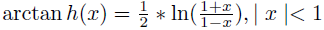Yes, these are exactly the same equations I presented above. Fisher transform demystified! Fisher transform is simply arctanh(x) and Inverse Fisher Transform is its inverse, tanh(x)!

### 6. Trading signals module

In order to verify the Inverse Fisher Transform I build a trading signal module based on Inverse Fisher Transform indicator.

You may find it useful to see the trading module based on a custom indicator. I used CiCustom class instance to hold Inverse Fisher indicator and overridden four virtual methods of CExpertSignal class: CheckOpenLong() and CheckOpenShort() are resposible for generating signals when there is no open position and CheckReverseLong() and CheckReverseShort() are responsible for reversing open position.

```//+------------------------------------------------------------------+
//|                               InverseFisherRSISmoothedSignal.mqh |
//|                                    Copyright © 2011, Investeo.pl |
//|                                               http://Investeo.pl |
//|                                                      Version v01 |
//+------------------------------------------------------------------+
#property tester_indicator "SmoothedRSIInverseFisherTransform.ex5"
//+------------------------------------------------------------------+
//| include files                                                    |
//+------------------------------------------------------------------+
#include <Expert\ExpertSignal.mqh>
//+------------------------------------------------------------------+
//| Class CSignalInverseFisherRSISmoothed.                           |
//| Description: Class generating InverseFisherRSISmoothed signals   |
//|              Derived from CExpertSignal.                         |
//+------------------------------------------------------------------+

// wizard description start
//+------------------------------------------------------------------+
//| Description of the class                                         |
//| Title=Signal on the Inverse Fisher RSI Smoothed Indicator        |
//| Type=SignalAdvanced                                              |
//| Name=InverseFisherRSISmoothed                                    |
//| Class=CSignalInverseFisherRSISmoothed                            |
//| Page=                                                            |
//+------------------------------------------------------------------+
// wizard description end
//+------------------------------------------------------------------+

//+------------------------------------------------------------------+
//| CSignalInverseFisherRSISmoothed class                            |
//| Purpose: A class of a module of trade signals,                   |
//| on InverseFisherRSISmoothed                                      |
//+------------------------------------------------------------------+
class CSignalInverseFisherRSISmoothed : public CExpertSignal
{
protected:
CiCustom          m_invfish;
double            m_stop_loss;

public:
CSignalInverseFisherRSISmoothed();
//--- methods initialize protected data
virtual bool      InitIndicators(CIndicators *indicators);
virtual bool      ValidationSettings();
//---
virtual bool      CheckOpenLong(double &price,double &sl,double &tp,datetime &expiration);
virtual bool      CheckReverseLong(double &price,double &sl,double &tp,datetime &expiration);
virtual bool      CheckOpenShort(double &price,double &sl,double &tp,datetime &expiration);
virtual bool      CheckReverseShort(double &price,double &sl,double &tp,datetime &expiration);

protected:
bool              InitInvFisher(CIndicators *indicators);
double            InvFish(int ind) { return(m_invfish.GetData(0,ind)); }
};
//+------------------------------------------------------------------+
//| Constructor CSignalInverseFisherRSISmoothed.                                    |
//| INPUT:  no.                                                      |
//| OUTPUT: no.                                                      |
//| REMARK: no.                                                      |
//+------------------------------------------------------------------+
void CSignalInverseFisherRSISmoothed::CSignalInverseFisherRSISmoothed()
{
//--- initialize protected data
}
//+------------------------------------------------------------------+
//| Validation settings protected data.                              |
//| INPUT:  no.                                                      |
//| OUTPUT: true-if settings are correct, false otherwise.           |
//| REMARK: no.                                                      |
//+------------------------------------------------------------------+
bool CSignalInverseFisherRSISmoothed::ValidationSettings()
{
//--- initial data checks
if(!CExpertSignal::ValidationSettings()) return(false);
//--- ok
return(true);
}

//+------------------------------------------------------------------+
//| Create Inverse Fisher custom indicator.                          |
//| INPUT:  indicators -pointer of indicator collection.             |
//| OUTPUT: true-if successful, false otherwise.                     |
//| REMARK: no.                                                      |
//+------------------------------------------------------------------+
bool CSignalInverseFisherRSISmoothed::InitInvFisher(CIndicators *indicators)
{
//--- check pointer
printf(__FUNCTION__+": initializing Inverse Fisher Indicator");
if(indicators==NULL) return(false);
//--- add object to collection
if(!indicators.Add(GetPointer(m_invfish)))
{
printf(__FUNCTION__+": error adding object");
return(false);
}
MqlParam invfish_params[];
ArrayResize(invfish_params,2);
invfish_params.type=TYPE_STRING;
invfish_params.string_value="SmoothedRSIInverseFisherTransform";
//--- applied price
invfish_params.type=TYPE_INT;
invfish_params.integer_value=PRICE_CLOSE;
//--- initialize object
if(!m_invfish.Create(m_symbol.Name(),m_period,IND_CUSTOM,2,invfish_params))
{
printf(__FUNCTION__+": error initializing object");
return(false);
}
m_invfish.NumBuffers(18);
//--- ok
return(true);
}
//+------------------------------------------------------------------+
//| Create indicators.                                               |
//| INPUT:  indicators -pointer of indicator collection.             |
//| OUTPUT: true-if successful, false otherwise.                     |
//| REMARK: no.                                                      |
//+------------------------------------------------------------------+
bool CSignalInverseFisherRSISmoothed::InitIndicators(CIndicators *indicators)
{
//--- check pointer
if(indicators==NULL) return(false);
//--- initialization of indicators and timeseries of additional filters
if(!CExpertSignal::InitIndicators(indicators)) return(false);
//--- create and initialize SAR indicator
if(!InitInvFisher(indicators)) return(false);
m_stop_loss = 0.0010;
//--- ok
printf(__FUNCTION__+": all inidicators properly initialized.");
return(true);
}
//+------------------------------------------------------------------+
//| Check conditions for long position open.                         |
//| INPUT:  price      - reference for price,                        |
//|         sl         - reference for stop loss,                    |
//|         tp         - reference for take profit,                  |
//|         expiration - reference for expiration.                   |
//| OUTPUT: true-if condition performed, false otherwise.            |
//| REMARK: no.                                                      |
//+------------------------------------------------------------------+
bool CSignalInverseFisherRSISmoothed::CheckOpenLong(double &price,double &sl,double &tp,datetime &expiration)
{
printf(__FUNCTION__+" checking signal");

int idx=StartIndex();

//---
price=0.0;
tp   =0.0;
//---
if(InvFish(idx+2)<12.0 && InvFish(idx+1)>12.0)
{
printf(__FUNCTION__ + " BUY SIGNAL");
return true;
} else printf(__FUNCTION__ + " NO SIGNAL");
//---
return false;
}
//+------------------------------------------------------------------+
//| Check conditions for long position close.                        |
//| INPUT:  price - refernce for price.                              |
//| OUTPUT: true-if condition performed, false otherwise.            |
//| REMARK: no.                                                      |
//+------------------------------------------------------------------+
bool CSignalInverseFisherRSISmoothed::CheckReverseLong(double &price,double &sl,double &tp,datetime &expiration)
{
long tickCnt;
int ticks=CopyTickVolume(Symbol(), 0, 0, 1, tickCnt);
if (ticks!=1 || tickCnt!=1) return false;

int idx=StartIndex();

price=0.0;
// sl   =m_symbol.NormalizePrice(m_symbol.Bid()+20*m_stop_level);
//---

if((InvFish(idx+1)>88.0 && InvFish(idx)<88.0)  ||
(InvFish(idx+2)>88.0 && InvFish(idx+1)<88.0) ||
(InvFish(idx+2)>12.0 && InvFish(idx+1)<12.0))
{
printf(__FUNCTION__ + " REVERSE LONG SIGNAL");
return true;
} else printf(__FUNCTION__ + " NO SIGNAL");
return false;
}
//+------------------------------------------------------------------+
//| Check conditions for short position open.                        |
//| INPUT:  price      - refernce for price,                         |
//|         sl         - refernce for stop loss,                     |
//|         tp         - refernce for take profit,                   |
//|         expiration - refernce for expiration.                    |
//| OUTPUT: true-if condition performed, false otherwise.            |
//| REMARK: no.                                                      |
//+------------------------------------------------------------------+
bool CSignalInverseFisherRSISmoothed::CheckOpenShort(double &price,double &sl,double &tp,datetime &expiration)
{
printf(__FUNCTION__+" checking signal");
int idx=StartIndex();
//---
price=0.0;
sl   = 0.0;
//---
if(InvFish(idx+2)>88.0 && InvFish(idx+1)<88.0)
{printf(__FUNCTION__ + " SELL SIGNAL");
return true;} else printf(__FUNCTION__ + " NO SIGNAL");

//---
return false;
}
//+------------------------------------------------------------------+
//| Check conditions for short position close.                       |
//| INPUT:  price - refernce for price.                              |
//| OUTPUT: true-if condition performed, false otherwise.            |
//| REMARK: no.                                                      |
//+------------------------------------------------------------------+
bool CSignalInverseFisherRSISmoothed::CheckReverseShort(double &price,double &sl,double &tp,datetime &expiration)
{
long tickCnt;
int ticks=CopyTickVolume(Symbol(), 0, 0, 1, tickCnt);
if (ticks!=1 || tickCnt!=1) return false;

int idx=StartIndex();

price=0.0;
//---

if((InvFish(idx+1)<12.0 && InvFish(idx)>12.0) ||
(InvFish(idx+2)<12.0 && InvFish(idx+1)>12.0) ||
(InvFish(idx+2)<88.0 && InvFish(idx+1)>88.0))
{
printf(__FUNCTION__ + " REVERSE SHORT SIGNAL");
return true;
} else printf(__FUNCTION__ + " NO SIGNAL");
return false;
}
```

### 7. Expert Advisor

In order to verify the Inverse Fisher Transform I build a standard EA that uses the trading signal module presented earlier.

I also added trailing stop-loss module taken from the article "MQL5 Wizard: How to Create a Module of Trailing of Open Positions".

```//+------------------------------------------------------------------+
//|                                                 InvRSIFishEA.mq5 |
//|                        Copyright 2011, MetaQuotes Software Corp. |
//|                                              https://www.mql5.com |
//+------------------------------------------------------------------+
#property copyright "Copyright 2011, MetaQuotes Software Corp."
#property link      "https://www.mql5.com"
#property version   "1.00"
//+------------------------------------------------------------------+
//| Include                                                          |
//+------------------------------------------------------------------+
#include <Expert\Expert.mqh>
//--- available signals
#include <Expert\Signal\MySignal\InverseFisherRSISmoothedSignal.mqh>
//--- available trailing
#include <Expert\Trailing\SampleTrailing.mqh>
//--- available money management
#include <Expert\Money\MoneyFixedLot.mqh>
//+------------------------------------------------------------------+
//| Inputs                                                           |
//+------------------------------------------------------------------+
//--- inputs for expert
input string Expert_Title         ="InvRSIFishEA";   // Document name
ulong        Expert_MagicNumber   =7016; //
bool         Expert_EveryTick     =true; //
//--- inputs for main signal
input int    Signal_ThresholdOpen =10;    // Signal threshold value to open [0...100]
input int    Signal_ThresholdClose=10;    // Signal threshold value to close [0...100]
input double Signal_PriceLevel    =0.0;   // Price level to execute a deal
input double Signal_StopLevel     =0.0;   // Stop Loss level (in points)
input double Signal_TakeLevel     =0.0;   // Take Profit level (in points)
input int    Signal_Expiration    =0;    // Expiration of pending orders (in bars)
input double Signal__Weight       =1.0;   // InverseFisherRSISmoothed Weight [0...1.0]
//--- inputs for money
input double Money_FixLot_Percent =10.0;  // Percent
input double Money_FixLot_Lots    =0.2;   // Fixed volume
//+------------------------------------------------------------------+
//| Global expert object                                             |
//+------------------------------------------------------------------+
CExpert ExtExpert;
//+------------------------------------------------------------------+
//| Initialization function of the expert                            |
//+------------------------------------------------------------------+
int OnInit()
{
//--- Initializing expert
if(!ExtExpert.Init(Symbol(),Period(),Expert_EveryTick,Expert_MagicNumber))
{
//--- failed
printf(__FUNCTION__+": error initializing expert");
ExtExpert.Deinit();
return(-1);
}
//--- Creating signal
CSignalInverseFisherRSISmoothed *signal=new CSignalInverseFisherRSISmoothed;
if(signal==NULL)
{
//--- failed
printf(__FUNCTION__+": error creating signal");
ExtExpert.Deinit();
return(-2);
}
//---
ExtExpert.InitSignal(signal);
signal.ThresholdOpen(Signal_ThresholdOpen);
signal.ThresholdClose(Signal_ThresholdClose);
signal.PriceLevel(Signal_PriceLevel);
signal.StopLevel(Signal_StopLevel);
signal.TakeLevel(Signal_TakeLevel);
signal.Expiration(Signal_Expiration);

//--- Creation of trailing object
CSampleTrailing *trailing=new CSampleTrailing;
trailing.StopLevel(0);
trailing.Profit(20);

if(trailing==NULL)
{
//--- failed
printf(__FUNCTION__+": error creating trailing");
ExtExpert.Deinit();
return(-4);
}
//--- Add trailing to expert (will be deleted automatically))
if(!ExtExpert.InitTrailing(trailing))
{
//--- failed
printf(__FUNCTION__+": error initializing trailing");
ExtExpert.Deinit();
return(-5);
}
//--- Set trailing parameters
//--- Creation of money object
CMoneyFixedLot *money=new CMoneyFixedLot;
if(money==NULL)
{
//--- failed
printf(__FUNCTION__+": error creating money");
ExtExpert.Deinit();
return(-6);
}
//--- Add money to expert (will be deleted automatically))
if(!ExtExpert.InitMoney(money))
{
//--- failed
printf(__FUNCTION__+": error initializing money");
ExtExpert.Deinit();
return(-7);
}
//--- Set money parameters
money.Percent(Money_FixLot_Percent);
money.Lots(Money_FixLot_Lots);
//--- Check all trading objects parameters
if(!ExtExpert.ValidationSettings())
{
//--- failed
ExtExpert.Deinit();
return(-8);
}
//--- Tuning of all necessary indicators
if(!ExtExpert.InitIndicators())
{
//--- failed
printf(__FUNCTION__+": error initializing indicators");
ExtExpert.Deinit();
return(-9);
}
//--- ok
return(0);
}
//+------------------------------------------------------------------+
//| Deinitialization function of the expert                          |
//+------------------------------------------------------------------+
void OnDeinit(const int reason)
{
ExtExpert.Deinit();
}
//+------------------------------------------------------------------+
//| "Tick" event handler function                                    |
//+------------------------------------------------------------------+
void OnTick()
{
ExtExpert.OnTick();
}
//+------------------------------------------------------------------+
//| "Trade" event handler function                                   |
//+------------------------------------------------------------------+
void OnTrade()
{
ExtExpert.OnTrade();
}
//+------------------------------------------------------------------+
//| "Timer" event handler function                                   |
//+------------------------------------------------------------------+
void OnTimer()
{
ExtExpert.OnTimer();
}
//+------------------------------------------------------------------+
```

I must admit that the EA was not profitable for every asset and for each timeframe but I tweaked it to give quite good results for EURUSD 1H timeframe.

I encourage readers to try to change the signal module and the indicator settings, you may find more profitable EA than presented in the article.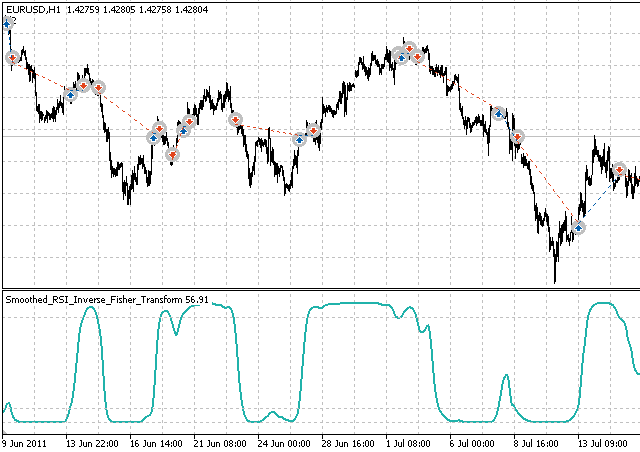Figure 10. Inverse Fisher Transform EA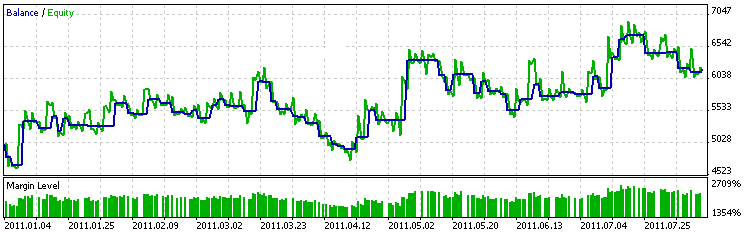Figure 11. Inverse Fisher Transform EA balance graph

### Conclusion

I hope that the article provided a good introduction to Fisher Transform and Inverse Fisher Transform and showed a way to build a signal trading module based on a custom indicator.

I used Sylvain's Vervoort Smoothed RSI Inverse Fisher Transform indicator but in fact you can easily apply Inverse Fisher Transform to any oscillator and build EA based on this article.

I also encourage readers to tweak the settings to make a profitable EAs based on the one I presented. I am providing external links for further reference below.

### References

1. The Fisher Transform
2. Using the Fisher Transform
3. The Inverse Fisher Transform
4. Smoothed RSI Inverse Fisher Transform

Attached files |

#### Other articles by this author

Last comments | Go to discussion (5)| 3 Sep 2011 at 22:22

The indicator works fine but when running the Expert Advisor, it gives out error messages and is not able to run. Please check. Thanks.

Here is copy of error messages:

2011.09.03 14:24:40 Core 1 2010.01.01 00:00:00   OnInit: error initializing indicators
2011.09.03 14:24:40 Core 1 2010.01.01 00:00:00   CExpert::InitIndicators: error initialization indicators of signal object
2011.09.03 14:24:40 Core 1 2010.01.01 00:00:00   CExpertBase::InitIndicators: parameters of setting are not checked
2011.09.03 14:24:40 Core 1 2010.01.01 00:00:00   CExpertBase::SetOtherSeries: changing of timeseries is forbidden
2011.09.03 14:24:40 Core 1 2010.01.01 00:00:00   CExpertBase::SetPriceSeries: changing of timeseries is forbidden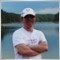| 5 Sep 2011 at 12:43
Kayos:

The indicator works fine but when running the Expert Advisor, it gives out error messages and is not able to run. Please check. Thanks.

Here is copy of error messages:

...
2011.09.03 14:24:40 Core 1 2010.01.01 00:00:00   CExpertBase::SetPriceSeries: changing of timeseries is forbidden

Do you use last build of MetaTrader5? Standard library has been changed some time ago.| 25 Nov 2011 at 07:08
Rosh:
Do you use last build of MetaTrader5? Standard library has been changed some time ago.

Latest Release, same problem.

Plz check. Tks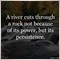| 29 Aug 2017 at 13:22

Can the file pleas be available in mql4, I will really appreciate it.

Thank you.| 4 Jun 2021 at 06:39
The article and the signals are very interesting but I notice that there are no more exchanges on the subject since years. I still try my luck with my question about the backtest that does not work. I think it's related to the error you see in the screenshot. If someone has an idea...Thanks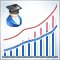Creating Custom Criteria of Optimization of Expert Advisors
The MetaTrader 5 Client Terminal offers a wide range of opportunities for optimization of Expert Advisor parameters. In addition to the optimization criteria included in the strategy tester, developers are given the opportunity of creating their own criteria. This leads to an almost limitless number of possibilities of testing and optimizing of Expert Advisors. The article describes practical ways of creating such criteria - both complex and simple ones.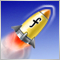3 Methods of Indicators Acceleration by the Example of the Linear Regression
The article deals with the methods of indicators computational algorithms optimization. Everyone will find a method that suits his/her needs best. Three methods are described here.One of them is quite simple, the next one requires solid knowledge of Math and the last one requires some wit. Indicators or MetaTrader5 terminal design features are used to realize most of the described methods. The methods are quite universal and can be used not only for acceleration of the linear regression calculation, but also for many other indicators.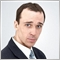Andrey Voitenko (avoitenko): &quot;Developers benefit from the ideas that they code? Nonsense!&quot;
A Ukrainian developer Andrey Voitenko (avoitenko) is an active participant of the "Jobs" service at mql5.com, helping traders from all over the world to implement their ideas. Last year Andrey's Expert Advisor was on the fourth place in the Automated Trading Championship 2010, being slightly behind the bronze winner. This time we are discussing the Jobs service with Andrey.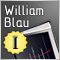William Blau's Indicators and Trading Systems in MQL5. Part 1: Indicators
The article presents the indicators, described in the book by William Blau "Momentum, Direction, and Divergence". William Blau's approach allows us to promptly and accurately approximate the fluctuations of the price curve, to determine the trend of the price movements and the turning points, and eliminate the price noise. Meanwhile, we are also able to detect the overbought/oversold states of the market, and signals, indicating the end of a trend and reversal of the price movement.